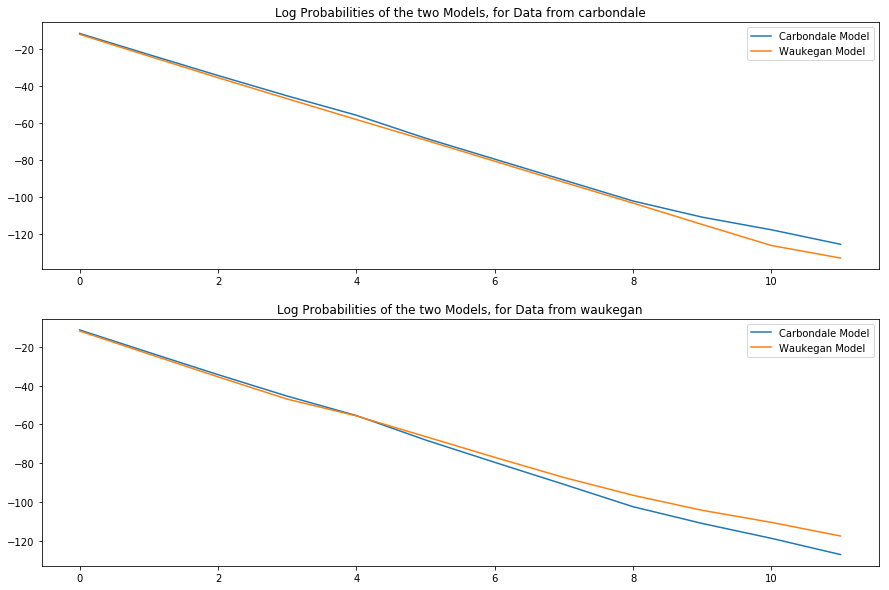# ECE 417¶

### Fall 2018, Lecture 12: Hidden Markov Models¶

This is an ipynb notebook, so the first thing I want to do is to load some libraries.

In :
import numpy as np
import matplotlib.pyplot as plt
import requests
%matplotlib inline


Now I'm going to download some data that I put on the course web page. These are temperature records (monthly high temperature, and monthly log temperature, in Fahrenheit) from two cities in the state of Illinois: Waukegan (in the far northeast corner), and Carbondale (in the far southern point of the state). The data originally came from here: https://www.isws.illinois.edu/data/climatedb/. I have extracted CSV files, which you might want to look at, for example, to see the dates over which they are collected: http://courses.engr.illinois.edu/ece417/fa2018/ps/carbondale_climate_edited.txt and http://courses.engr.illinois.edu/ece417/fa2018/ps/waukegan_climate_edited.txt

In :
URLformat = 'http://courses.engr.illinois.edu/ece417/fa2018/ps/%s_climate_edited.txt'
cities = ['carbondale','waukegan']
rawdata = {}
for city in [0,1]:
r = requests.get(URLformat % cities[city])
rawdata[city] = [ x.rstrip().split(',') for x in r.text.split('\n') ]
print(rawdata[city][0:3])

[['ï»¿YearÂ\xa0', 'MonthÂ\xa0', 'High', 'LowÂ'], ['2005', 'Jan.', '45.6', '31'], ['2005', 'Feb.', '50.3', '33.1']]
[['ï»¿YearÂ\xa0', 'MonthÂ\xa0', 'High', 'LowÂ'], ['1923', 'Jan.', '35.1', '20.3'], ['1923', 'Feb.', '29.5', '10.8']]


Now let's separate it into training data and testing data. Actually, this time, we won't use the training data at all, we'll use that next time. But we can look at some plots of the data, to see what type of data we have.

In :
months = ['Jan.','Feb.','Mar.','Apr.','May','Jun.','Jul.','Aug.','Sep.','Oct.','Nov.','Dec.']
train = [ np.zeros((0,2)), np.zeros((0,2)) ]  # train[y] is a 0x2 matrix
test = [ np.zeros((0,2)), np.zeros((0,2)) ]  # test[y] is a 0x2 matrix

for city in [0,1]:
for a in rawdata[city]:
if a in months and a.isdigit() and float(a)>-99:  # row gives one month, with temperature > -99
if len(test[city]) < 12:   # first twelve valid lines (first year's data) are the test data
test[city] = np.vstack((test[city],[float(a),float(a)]))
elif len(train[city]) < 72:          # next six years as training data
train[city] = np.vstack((train[city],[float(a),float(a)]))

fig, axs = plt.subplots(2,2,figsize=(15,5))
for city in [0,1]:
axs[city,0].plot(train[city])
axs[city,0].set_title('Train Data for %s'%cities[city])
axs[city,1].plot(test[city])
axs[city,1].set_title('Test Data for %s'%cities[city])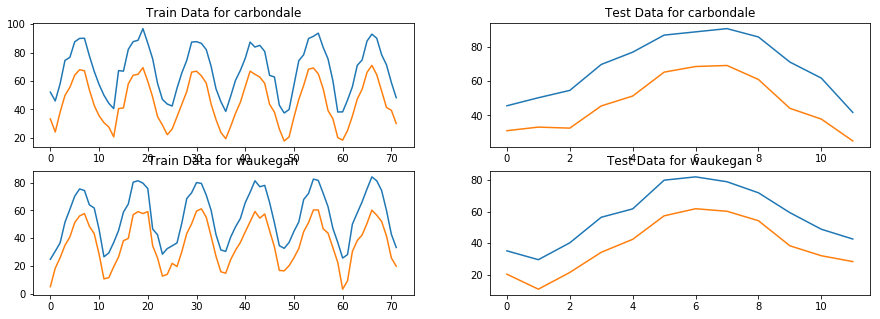Obviously, in any given season, Carbondale is warmer than Waukegan. Obviously, though, the two distributions overlap. If we have temperature measurements for a "mystery city" and want to know which city it is, then we need to know not just the average temperature --- we need to know the average temperature for each season. Suppose somebody gives us those averages, as follows:

In :
all_means = np.array([[[48,28],[78,56],[87,63],[58,35]],[[36,19],[65,43],[77,57],[46,29]]])
fig, axs = plt.subplots(1,2,figsize=(15,5))
for city in [0,1]:
axs[city].scatter(all_means[city,:,0],all_means[city,:,1])
axs[city].set_title('Seasonal Low vs High Temp, Averages for %s' % cities[city])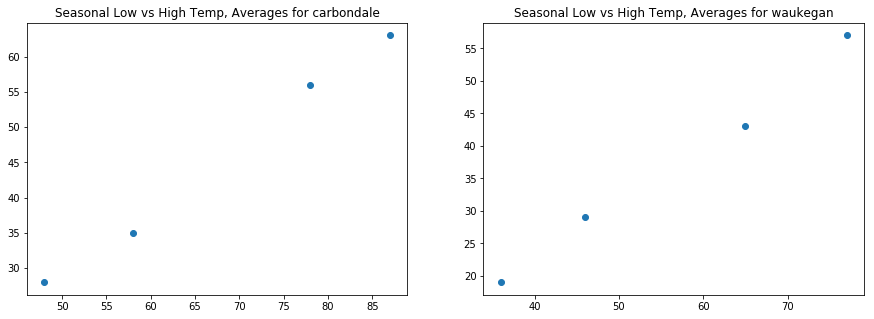So that's not quite enough. It will be more useful if somebody gives us the covariances. Here they are.

In :
all_covariances = np.array([[[[1323,1093],[1093,956]],
[[1111,1182],[1182,1319]],
[[475,436],[436,523]],
[[120,76],[76,55]]],
[[[959,963],[963,1110]],
[[1339,1089],[1089,948]],
[[422,273],[273,280]],
[[146,130],[130,119]]]])


The only useful way to plot covariances is by plotting the principal components.

In :
# Find the principal component directions and variances of each Gaussia
Lambda, V = np.linalg.eig(all_covariances)

# Let's show the mean vectors plus/minus each variance-scaled principal component direction
fig, axs = plt.subplots(1,2,figsize=(15,5))
for c in [0,1]:   # city
for s in [0,2]:  # pick out just two of the seasons: summer and winter
for m in [0,1]:      # principal component direction
x0 = all_means[c,s,0]+np.sqrt(Lambda[c,s,m])*np.array([-V[c,s,0,m],V[c,s,0,m]])
x1 = all_means[c,s,1]+np.sqrt(Lambda[c,s,m])*np.array([-V[c,s,1,m],V[c,s,1,m]])
axs[c].plot(x0,x1)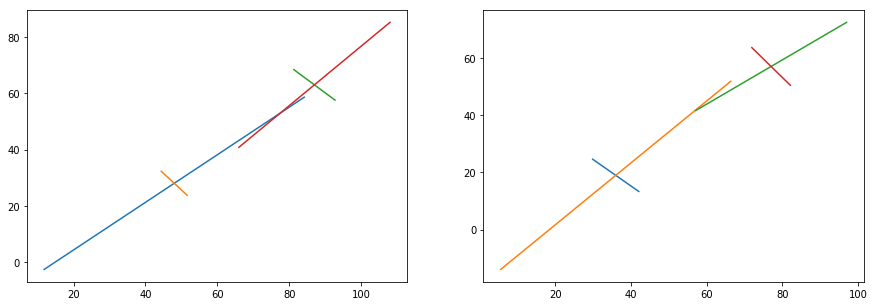Now somebody needs to give us the transition probabilities. We know that each year starts in Winter, then transitions (roughly) once every three months, and ends at the end of autumn (on December 21, which we'll call the end of the year). So the probability of transitioning out of a season is roughly 1/3, and the probability of staying in a season is roughly 2/3.

In :
A = np.array([[2/3,1/3,0,0],[0,2/3,1/3,0],[0,0,2/3,1/3],[0,0,0,1]])
A

Out:
array([[0.66666667, 0.33333333, 0.        , 0.        ],
[0.        , 0.66666667, 0.33333333, 0.        ],
[0.        , 0.        , 0.66666667, 0.33333333],
[0.        , 0.        , 0.        , 1.        ]])

Similarly, we know that every year starts in winter, so we can set the initial residence probabilities, pi, to be 1.0 for winter, and 0.0 for any other season.

In :
pi = np.array([1,0,0,0])
pi

Out:
array([1, 0, 0, 0])

Now let's define a function to calculate the Gaussian probability, given a mean and a covariance matrix.

In :
def gaussian(x,m,S):
det = np.linalg.det(S)
mahalanobis_squared = (x-m).dot(np.linalg.inv(S).dot(x-m))
return( np.exp(-0.5*mahalanobis_squared) / det)


For example, for each month in the test data, for Carbondale, let's calculate the log probability that the given month occurred in any given season.

In :
B = np.zeros((12,4))   # B[i,t] = log probability that month t, of the test data, occurred in season i
for t in range(0,12):
for i in range(0,4):
B[t,i]=np.log(gaussian(test[t,:],all_means[0,i,:],all_covariances[0,i,:,:]))
fig,axs=plt.subplots(1,1,figsize=(15,5))
axs.plot(B)
axs.legend(('Winter','Spring','Summer','Fall'))

Out:
<matplotlib.legend.Legend at 0x10efee0b8>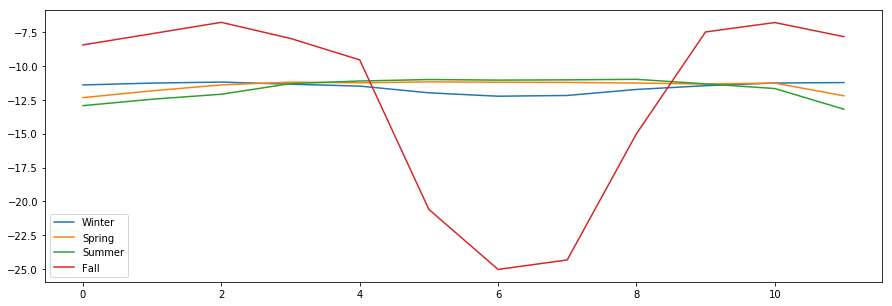OK, the low covariance of autumn temperatures may cause some trouble later on...

Let's write a function that will implement the forward algorithm. Given a full set of HMM parameters, it will compute the scaled alpha parameters, and the Gain terms, G.

In :
def compute_alpha_and_G(X, A, pi, means, covariances):
T = len(X[:,0])
N = len(pi)
alpha = np.zeros((T,N))
G = np.zeros((T))
sum_of_log_G = np.zeros((T))
#############################
# First frame
for i in range(0,N):
alpha[0,i] = pi[i]*gaussian(X[0,:],means[i,:],covariances[i,:,:])
G = np.sum(alpha[0,:])
alpha[0,:] /= G
sum_of_log_G = np.log(G)
################################
# All of the other frames
for t in range(1,T):
for j in range(0,N):
for i in range(0,N):
alpha[t][j] += alpha[t-1][i]*A[i,j]*gaussian(X[t,:],means[j,:],covariances[j,:,:])
G[t] = np.sum(alpha[t,:])
alpha[t,:] /= G[t]
sum_of_log_G[t] = sum_of_log_G[t-1] + np.log(G[t])
return alpha,G,sum_of_log_G


First, let's test it by finding the probabilities for the Carbondale, given the Carbondale model.

In :
alpha,G,sum_of_log_G = compute_alpha_and_G(test,A,pi,all_means,all_covariances)
fig,axs=plt.subplots(2,1,figsize=(15,10))
axs.plot(sum_of_log_G)
axs.set_title('Total log prob of Carbondale data, according to Carbondale model, as a function of month')
axs.plot(alpha)
axs.set_title('Normalized probability of each season, for each month of Carbondale data')
axs.legend(('Winter','Spring','Summer','Fall'))

Out:
<matplotlib.legend.Legend at 0x10eee8710>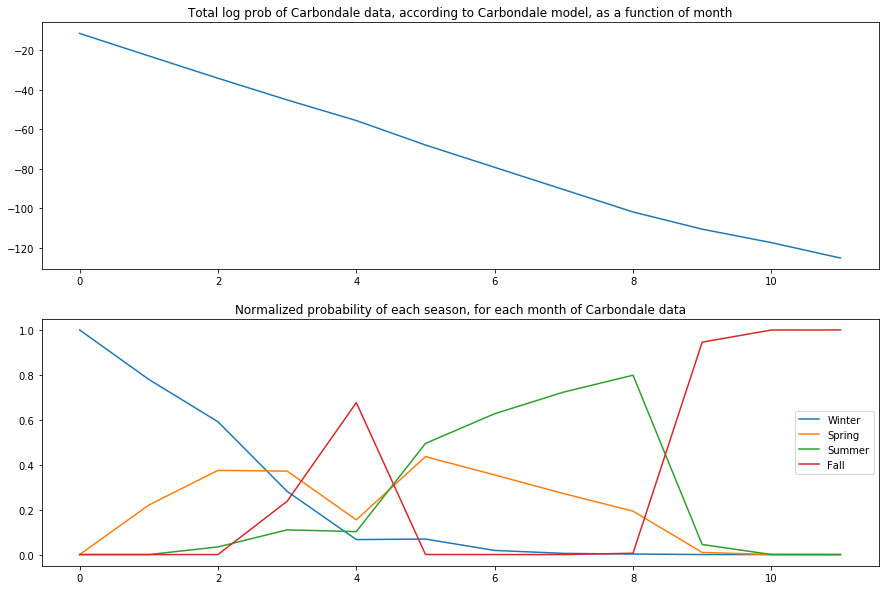### Testing¶

Now, for each of the two test tokens, let's find out how probable it is, according to each of the two possible city models.

In :
alphas = [0,0]
G_scores = [0,0]
prob_scores = [0,0]
for ref in [0,1]:    # reference label (the city the data really came from)
alphas[ref]=[0,0]
G_scores[ref]=[0,0]
prob_scores[ref]=[0,0]
X = test[ref]
for hyp in [0,1]:    # hypothesis (the model we are testing, to see how well it matches the data)
means = all_means[hyp]
covariances = all_covariances[hyp]
alphas[ref][hyp],G_scores[ref][hyp],prob_scores[ref][hyp]=compute_alpha_and_G(X,A,pi,means,covariances)

In :
fig,axs=plt.subplots(2,1,figsize=(15,10))
for ref in [0,1]:
axs[ref].plot(np.linspace(0,11,12),prob_scores[ref],np.linspace(0,11,12),prob_scores[ref])
axs[ref].set_title('Log Probabilities of the two Models, for Data from %s' % cities[ref])
axs[ref].legend(('Carbondale Model','Waukegan Model'))